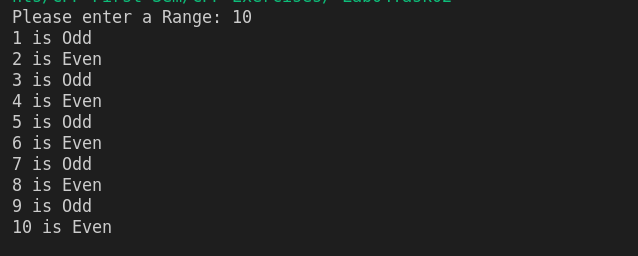Write a C++ program that asks a user to input the range (final value) up to which the loop should run and then for each number in that range, it should tell whether that (each) number is Odd or Even. For example, if the user enters 7 as the final value of the range, then it should display like follows:cpp programming exercise with solution

#include <iostream>
using namespace std;

int main()
{
int rang;
cin>>rang;
for (int i = 1; i<= rang; i++)
{
if (i % 2 == 0)
{
cout<<i<<" is Even"<<endl;
}
else
{
cout<<i<<" is Odd"<<endl;
}
}

return 0;
}

By using while loop

#include <iostream>
using namespace std;

int main()
{
int rang;
int i = 1;
cin>>rang;

while(i <= rang)
{
if (i % 2 == 0)
{
cout<<i<<" is Even"<<endl;
}
else
{
cout<<i<<" is Odd"<<endl;
}
i++;
}
return 0;
}